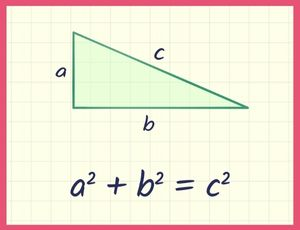Register for Maths, Science, English, Reasoning Olympiad Exams Register here | Book Free Demo for Live Olympiad Classes here | Check Olympiad Exam Dates here | Buy Practice Papers for IMO, IOM, HEO, IOEL etc here | Login here to participate in all India free mock test on every Saturday

# TheoremA result that has been proved to be true (using operations and facts that were already known). Example: The "Pythagoras Theorem" proved that a2 + b2 = c2 for a right angled triangle. Other examples: ? Intermediate Value Theorem ? Binomial Theorem ? Fund

70%